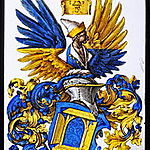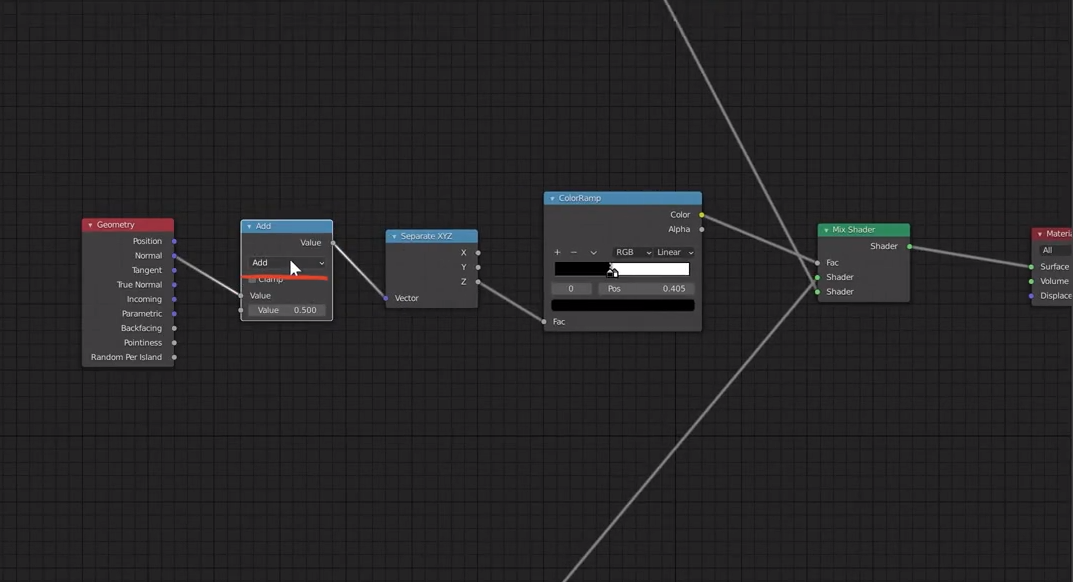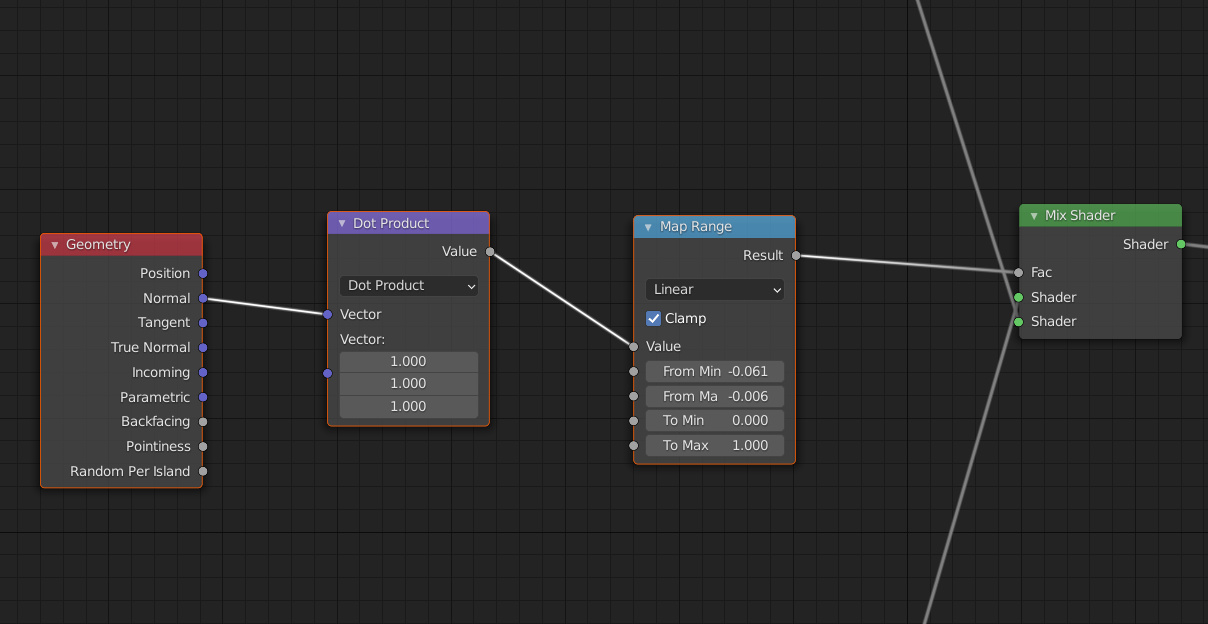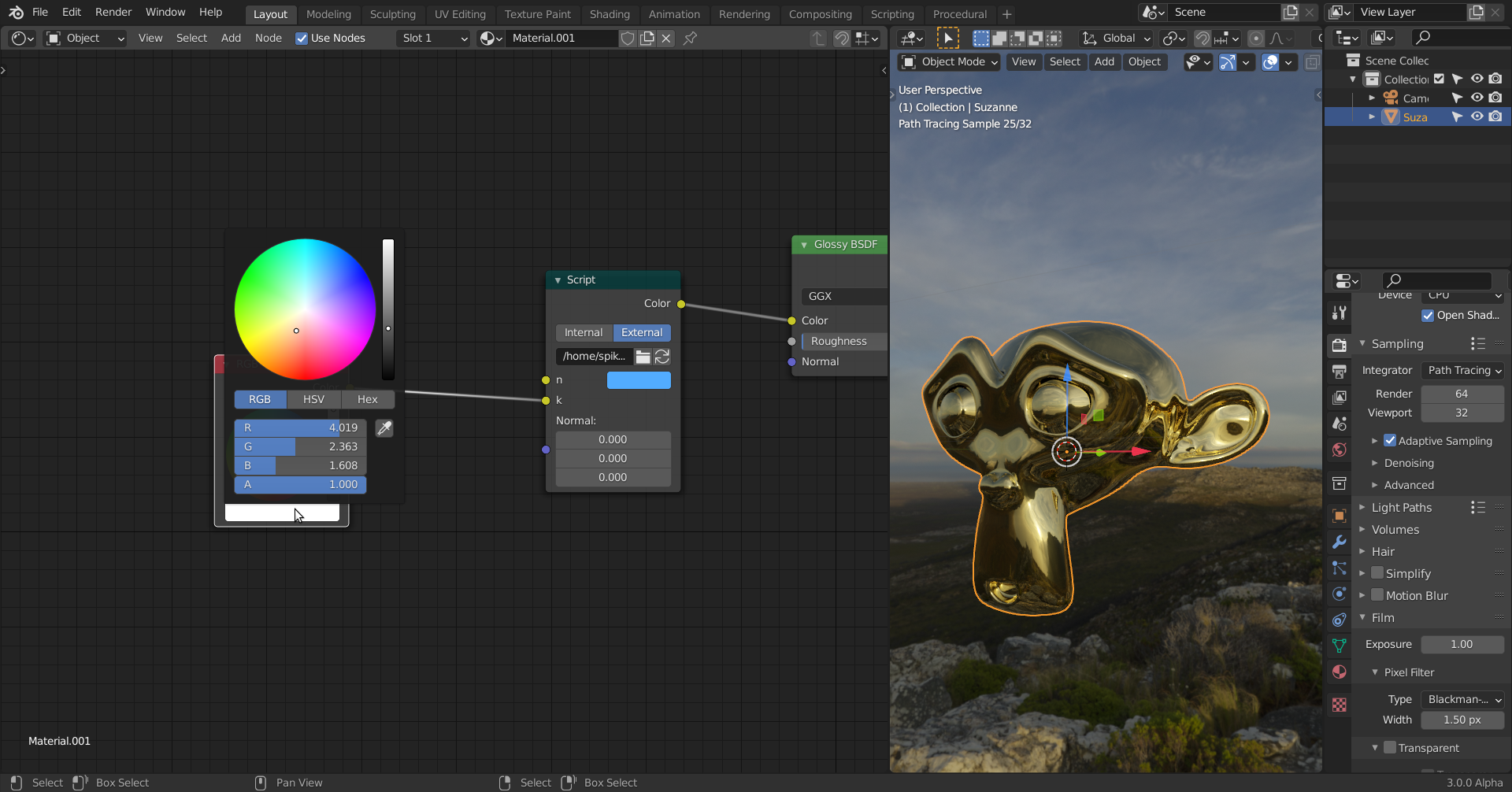135 replies · posted

## Spikey's Math Lab

Get it? Math Lab.... sounds like Me.... Lab?.... No? ok

Sospikeyxxx  you're like a super mathematician, I'm doing the Blender Cloud Procedural Shading course and it is super heavy dense in tough, difficult and impossible things and worst of all there's like a lot of math involved. I wanted to use this to bother you a lot and ask you math questions when it comes to procedural shading.

The course is good but Simon goes way too fast and doesn't get into the really basics and assumes you know what happens mathematically under the hood.

*WARNING, HERE BE MATH*

•spikeyxxx I have recently seen a node setup in the "Shader Editor" where someone plugged the "Normal" output socket of a "Geometry Node" into one of the two "Value" input sockets of a "Math" node (set to "Add"). Besides the question whether this makes sense I'm wondering how the vector is converted into a single number?

•duerer I would love to see that node setup! (Maybe it makes sense in some cases...)

Maybe that is a genius, but it is also possible, that this was done by someone who has no idea what he/she is doing ;)

I took me some time to figure out what is happening, because unlike when you plug a color into a value and Blender uses the Luminasnce of the color, when you plug a vector into a value, Blender takes the average of the X, Y and Z. Like this:But things get curiouser and curiouser; When you plug the Vector into a MixRGB Node first, then the output of that MixRGB Node is a vector, but when you plug that into a grey socket, Blender uses the Luminance formula on the X, Y  and Z values!

So these two flows are the same:• Thank you,spikeyxxx, for your answer 😀! The Blender 2.93 Manual here is unfortunately incomplete since it doesn't explain what conversion happens when plugging a "Vector" output socket into a "Value" input socket. I wish there would be a "Socket Output Display" node that you could connect to an output socket  so that it shows the value or the array of values that this socket provides.  This node should also have some checkboxes which you can choose in in order to show how this value is converted into other data types.

PS: This is the node setup I mentioned:Interestingly, I've learned in that context that a "Normal Map" node with default settings ("Tangent Space") and no "Normal Texture" plugged into it outputs the same result as the "Normal" output socket of a "Texture Coordinate" node.

•duerer that node setup is definitely not made by a genius ;)

Plugging a value (output from the Math Node) into a vector socket, means that the X, Y and Z of that vector all get that same value. To then separate the X, Y and Z and only use the Z....That person has absolutely no idea what he/she is doing.

Without the separate XYZ, it would be something like this:Both 'paths' give the exact same result. I muted the Add node and subtracted 0.5 from the 'From Min' and 'From Max' in the Map Range (which you cannot do with the ColorRamp).

In words: the creator of this node setup has made an (imaginary) diagonal plane (which has a Normal Vector pointing in the direction (1, 1, 1)) and looks at how close the Normal of each polygon of the Object that uses this shader, is to that Vector (1, 1, 1). And all this in, what I would call, a clumsy and very unintelligible way...

• And here is how I would do it:•spikeyxxx One question to your last image in your post here (with an orange frame): At the "MixRGB" node (set to "Add") you put a label that the output vector still has "negative values". But since it's a "Color Output" socket, isn't it clamped to the interval [0, 1]?

In the node setup screenshot that I've posted above, I think that the guy who has created this, maybe just by habit used the "Math" node instead of the "Vector" Math" node since the first one, at least to my perception, is more often used in tutorials than the second one (oh, a pun 😉!). With a "Vector Math" node, the "Separate XYZ" node would really just isolate the Z-component.

• But since it's a "Color Output" socket, isn't it clamped to the interval [0, 1]?

No, but that is what you would expect, so that is why I explicitly mention that.

Simple example, showing this:And not:With a "Vector Math" node, the "Separate XYZ" node would really just isolate the Z-component.

Yes, but he puts a value (from the Math Node) into a Vector socket, turning it into a vector with three the same values and then he uses one of them....So, like: a is a number, then make a vector (a, a, a) and then consider the third position of that vector (the Z)..

Personally, I think that is stupid ;)

•spikeyxxx That's really strange that the manual setting of a color only allows values from 0 to 1 for R, G and B but if you take the values from a vector it allows negative values:• But actually, the upper bound of 1 is a soft limit; you can manually type in a number higher than 1. (But not below 0.) This is for instance useful when using 'complex' Fresnel with metals: